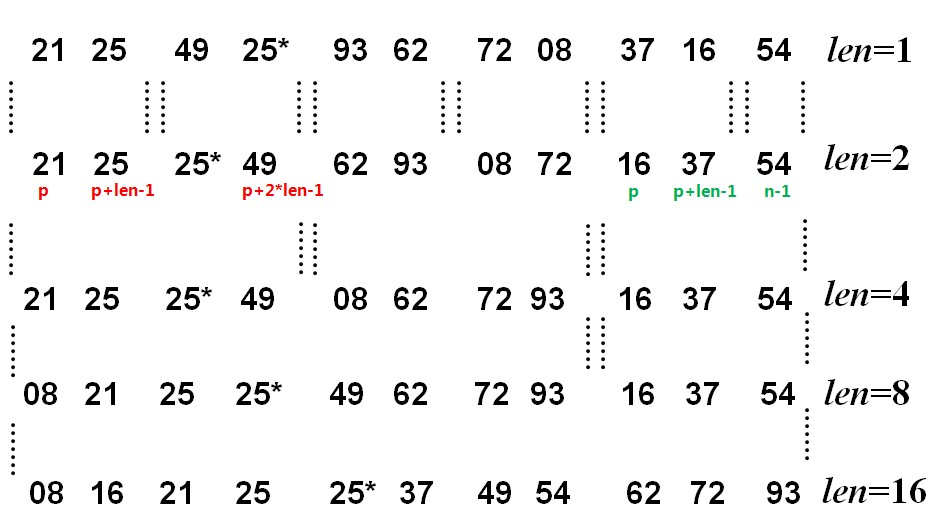﻿ 关于Java排序算法-归并排序（Merge Sort） – 蒋智昊的博客# 关于Java排序算法-归并排序（Merge Sort）```public class MergeSort {
public static void main(String[] args) {
//int[] arr = { 9, 8, 7, 6, 5, 4, 3, 2, 1 };
int[] arr = { 21, 25, 49, 25, 93, 62, 72, 8, 37, 16, 54 };

display("原始数据: ", arr);

mainMerge(arr, arr.length);

display("排序结果: ", arr);
}

// 显示
public static void display(String str, int[] arr) {
System.out.println(str);
for (int i = 0; i < arr.length; i++) {
System.out.print(arr[i] + " ");
}
System.out.println();
}

// 归并排序主算法：
// 第一趟: arr归并到arrDest；
// 第二趟: arrDest归并到arr； ......，需偶数趟。
// 若最后一趟 len>=n，则arrDest直接复制到arr。
public static void mainMerge(int[] arr, int n) {
// 对数组arr[n]中的记录进行归并排序
int[] arrDest = new int[n];
int len = 1;
while (len < n) {
oneMerge(arr, arrDest, n, len);
len *= 2;
oneMerge(arrDest, arr, n, len);
len *= 2;
}
// 清空临时数组
arrDest = null;
}

// 一趟归并排序算法：把arr[n]中长度为len的有序表两两归并到arrDest[n]
public static void oneMerge(int[] arr, int[] arrDest, int n, int len) {
int p = 0; // 一对待合并表的第一个元素的位置

// 循环两两归并
while (p + 2 * len - 1 <= n - 1) {
// twoMerge(int[] arr, int[] arrDest, int s, int m, int t)
// 将有序表arr[s..m] 和  arr[m+1..t] 归并为有序表arrDest[s..t]
twoMerge(arr, arrDest, p, p + len - 1, p + 2 * len - 1);
p += 2 * len;
}

if (p + len <= n - 1) {
// 归并最后两个长度不等的有序表
twoMerge(arr, arrDest, p, p + len - 1, n - 1);
} else {
// 复制最后剩下的一个有序表
for (int i = p; i <= n - 1; i++) {
arrDest[i] = arr[i];
}
}
}

// 二路归并算法：将有序表 arr[s..m] 和 arr[m+1..t] 归并为有序表arrDest[s..t]
public static void twoMerge(int[] arr, int[] arrDest, int s, int m, int t) {
int i, j, k;
i = s;
j = m + 1;
k = s; // 分别指向每个表的起始位置

// 两个有序表的归并
while (i <= m && j <= t) {
if (arr[i] <= arr[j]) {
arrDest[k] = arr[i];
i++;
k++;
} else {
arrDest[k] = arr[j];
j++;
k++;
}
}

// 复制第一个有序表中未归并的元素
while (i <= m) {
arrDest[k] = arr[i];
i++;
k++;
}

// 复制第二个有序表中未归并的元素
while (j <= t) {
arrDest[k] = arr[j];
j++;
k++;
}
}

}```